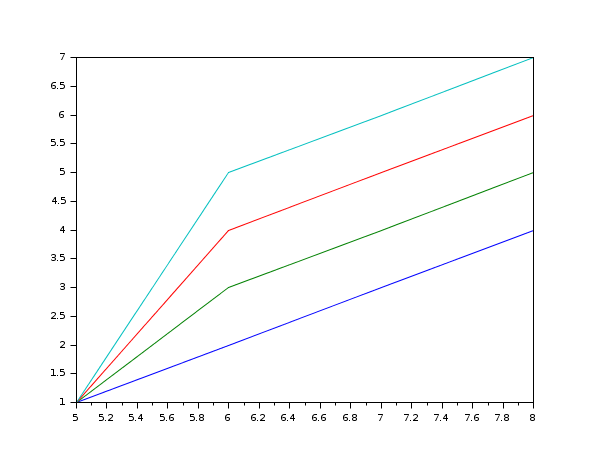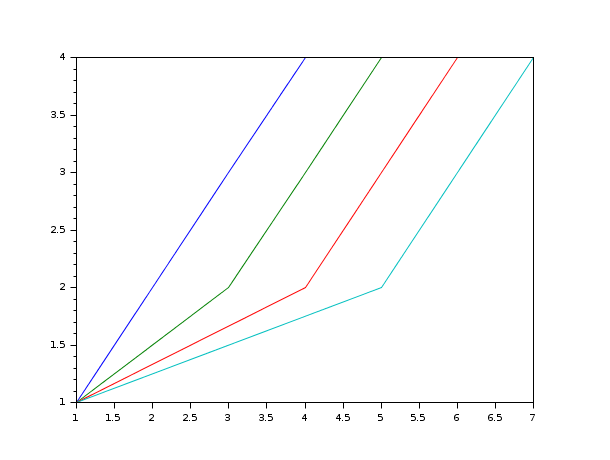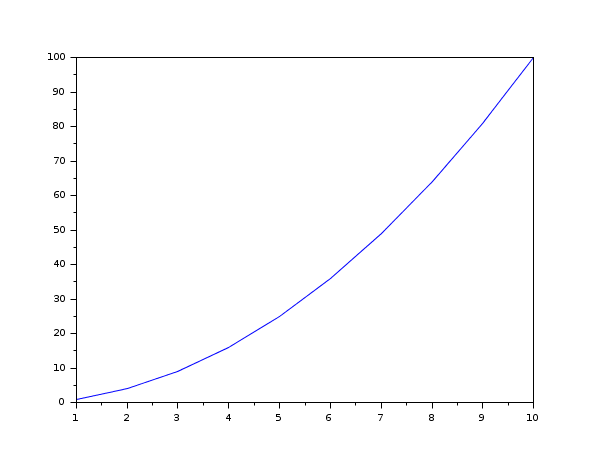Scilab Home page | Wiki | Bug tracker | Forge | Mailing list archives | ATOMS | File exchange
Change language to: English - Français - Português - 日本語 -

See the recommended documentation of this function

Справка Scilab >> Графики > 2d_plot > plot

plot

2D plot

Calling Sequence

```plot(y,<LineSpec>,<GlobalProperty>)
plot(x,y,<LineSpec>,<GlobalProperty>)
plot(x1,y1,<LineSpec1>,x2,y2,<LineSpec2>,...xN,yN,<LineSpecN>,<GlobalProperty1>,<GlobalProperty2>,..<GlobalPropertyM>)
plot(<axes_handle>,...)```

Arguments

x

a real matrix or vector. If omitted, it is assumed to be the vector `1:n` where `n` is the number of curve points given by the `y` parameter.

y

a real matrix or vector. `y` can also be a function defined as a macro or a primitive.

<LineSpec>

This optional argument must be a string that will be used as a shortcut to specify a way of drawing a line. We can have one `LineSpec` per `y` or `{x,y}` previously entered. `LineSpec` options deals with LineStyle, Marker and Color specifiers (see LineSpec). Those specifiers determine the line style, mark style and color of the plotted lines.

<GlobalProperty>

This optional argument represents a sequence of couple statements `{PropertyName,PropertyValue}` that defines global objects' properties applied to all the curves created by this plot. For a complete view of the available properties (see GlobalProperty).

<axes_handle>

This optional argument forces the plot to appear inside the selected axes given by `axes_handle` rather than the current axes (see gca).

Description

`plot` plots a set of 2D curves. `plot` has been rebuild to better handle Matlab syntax. To improve graphical compatibility, Matlab users should use `plot` (rather than plot2d).

Data entry specification :

In this paragraph and to be more clear, we won't mention `LineSpec` nor `GlobalProperty` optional arguments as they do not interfer with entry data (except for `"Xdata"`, `"Ydata"` and `"Zdata"` property, see GlobalProperty). It is assumed that all those optional arguments could be present too.

If `y` is a vector, plot(y) plots vector `y` versus vector `1:size(y,'*')`.

If `y` is a matrix, plot(y) plots each columns of `y` versus vector `1:size(y,1)`.

If `x` and `y` are vectors, plot(x,y) plots vector `y` versus vector `x`. `x` and `y` vectors should have the same number of entries.

If `x` is a vector and `y` a matrix plot(x,y) plots each columns of `y` versus vector `x`. In this case the number of columns of `y` should be equal to the number of `x` entries.

If `x` and `y` are matrices, plot(x,y) plots each columns of `y` versus corresponding column of `x`. In this case the `x` and `y` sizes should be the same.

Finally, if only `x` or `y` is a matrix, the vector is plotted versus the rows or columns of the matrix. The choice is made depending on whether the vector's row or column dimension matches the matrix row or column dimension. In case of a square matrix (on `x` or `y` only), priority is given to columns rather than lines (see examples below).When it is necessary and possible, `plot` transposes `x` and `y`, to get compatible dimensions; a warning is then issued. For instance, when `x` has as many rows as `y` has columns. If `y` is square, it is never transposed.

`y` can also be a function defined as a macro or a primitive. In this case, `x` data must be given (as a vector or matrix) and the corresponding computation `y(x)` is done implicitly.

The `LineSpec` and `GlobalProperty` arguments sould be used to customize the plot. Here is a complete list of the available options.

LineSpec

This option may be used to specify, in a short and easy manner, how the curves are drawn. It must always be a string containing references to LineStyle, Marker and Color specifiers.

These references must be set inside the string (order is not important) in an unambiguous way. For example, to specify a red long-dashed line with the diamond mark enabled, you can write : `'r--d'` or `'--dire'` or `'--reddiam'` or another unambiguous statement... or to be totally complete `'diamondred--'` (see LineSpec).

Note that the line style and color, marks color (and sizes) can also be (re-)set through the polyline entity properties (see polyline_properties).

GlobalProperty

This option may be used to specify how all the curves are plotted using more option than via `LineSpec`. It must always be a couple statement constituted of a string defining the `PropertyName`, and its associated value `PropertyValue` (which can be a string or an integer or... as well depending on the type of the `PropertyName`). Using `GlobalProperty`, you can set multiple properties : every properties available via LineSpec and more : the marker color (foreground and background), the visibility, clipping and thickness of the curves. (see GlobalProperty)

Note that all these properties can be (re-)set through the polyline entity properties (see polyline_properties).

Remarks

By default, successive plots are superposed. To clear the previous plot, use `clf()`. To enable `auto_clear` mode as the default mode, edit your default axes doing:

da=gda();

da.auto_clear = 'on'

For a better display `plot` function may modify the `box` property of its parent Axes. This happens when the parent Axes were created by the call to `plot` or were empty before the call. If one of the axis is centered at origin, the box is disabled. Otherwise, the box is enabled.

A default color table is used to color plotted curves if you do not specify a color. When drawing multiple lines, the plot command automatically cycles through this table. Here are the used colors:

 `R` `G` `B` 0. 0. 1. 0. 0.5 0. 1. 0. 0. 0. 0.75 0.75 0.75 0. 0.75 0.75 0.75 0. 0.25 0.25 0.25

Enter the command `plot` to see a demo.

Examples

```// x initialisation
x=[0:0.1:2*%pi]';
//simple plot
plot(sin(x))``````clf()
x=[0:0.1:2*%pi]';
plot(x,sin(x))``````clf()
//multiple plot
x=[0:0.1:2*%pi]';
plot(x,[sin(x) sin(2*x) sin(3*x)])``````clf()
x=[0:0.1:2*%pi]';
// axis on the right
plot(x,sin(x))
a=gca(); // Handle on current axes entity
a.y_location ="right";
clf()``````x=[0:0.1:2*%pi]';
// axis centered at (0,0)
plot(x-4,sin(x),x+2,cos(x))
a=gca(); // Handle on axes entity
a.x_location = "origin";
a.y_location = "origin";

// Some operations on entities created by plot ...
a=gca();
a.isoview='on';
a.children // list the children of the axes : here it is an Compound child composed of 2 entities
poly1= a.children.children(2); //store polyline handle into poly1
poly1.foreground = 4; // another way to change the style...
poly1.thickness = 3;  // ...and the tickness of a curve.
poly1.clip_state='off' // clipping control
a.isoview='off';``````//LineSpec and GlobalProperty examples:
clf();
t=0:%pi/20:2*%pi;
plot(t,sin(t),'ro-.',t,cos(t),'cya+',t,abs(sin(t)),'--mo')
scf(2)
plot([t ;t],[sin(t) ;cos(t)],'xdat',[1:2])
scf(3)
axfig3 = gca();
scf(4) // should remain blank
plot(axfig3,[t ;t],[sin(t) ;cos(t)],'zdat',[1:2],'marker','d','markerfac','green','markeredg','yel')
xdel(winsid())``````//Data specification
t=-%pi:0.1:%pi;
size(t)
plot(t) // simply plots y versus t vector size``````clf(); // clear figure
t=-%pi:0.1:%pi;
plot(t,sin(t)); // plots sin(t) versus t``````clf();
t=[1     1     1     1
2     3     4     5
3     4     5     6
4     5     6     7];

plot(t) // plots each t column versus row size``````clf();
t=[1     1     1     1
2     3     4     5
3     4     5     6
4     5     6     7];

subplot(221)
plot(t,sin(t)); // plots sin(t) versus t column by column this time
xtitle("sin(t) versus t")
subplot(222)
plot(t,sin(t)')
xtitle("sin(t)'' versus t")
subplot(223)
plot(t',sin(t))
a=gca();
a.data_bounds=[0 -1;7 1]; // to see the vertical line hiddden by the y axis
xtitle("sin(t) versus t''")
subplot(224)
plot(t',sin(t)')
xtitle("sin(t)'' versus t''")``````clf();
t=[1     1     1     1
2     3     4     5
3     4     5     6
4     5     6     7];

//Special case 1
//x : vector ([5 6 7 8]) and y : matrix (t)
x=[5 6 7 8]
plot(x,t);
plot(x',t); // idem, x is automatically transposed to match t (here the columns)``````clf()
x=[5 6 7 8]

t=[1     1     1     1
2     3     4     5
3     4     5     6
4     5     6     7];

// Only one matching possibility case: how to make 4 identical plots in 4 manners...
// x is 1x4 (vector) and y is 4x5 (non square matrix)
subplot(221);
plot(x,[t [8;9;10;12]]');
subplot(222);
plot(x',[t [8;9;10;12]]);
subplot(223);
plot(x,[t [8;9;10;12]]');
subplot(224);
plot(x',[t [8;9;10;12]]');``````clf()

t=[1     1     1     1
2     3     4     5
3     4     5     6
4     5     6     7];

//Special case 2
// Case where only x or y is a square matrix
//x : matrix (t) and y  : vector ([1 2 3 4])
plot(t,[1 2 3 4]') // equivalent to plot(t,[1 1 1 1;2 2 2 2;3 3 3 3;4 4 4 4])
plot(t,[1;2;3;4]') // the same plot, but here Y needs to be transposed``````t=[1     1     1     1
2     3     4     5
3     4     5     6
4     5     6     7];
clf();
cols = 1:4;

// cols is transposed : notice the priority given to the columns treatment
plot(t', cols) // equivalent to plot(t',[1 1 1 1;2 2 2 2;3 3 3 3;4 4 4 4])
plot(t', cols') // the same plot``````clf();
// y is a function defined by..
// ..a primitive
plot(1:0.1:10,sin) // equivalent to plot(1:0.1:10,sin(1:0.1:10))``````clf();
// ..a macro:
deff('[y]=toto(x)','y=x.*x')
plot(1:10,toto)```• plot2d — 2D plot
• surf — 3D surface plot
• scf — set the current graphic figure (window)
• clf — Clear or reset or reset a figure or a frame uicontrol.
• xdel — удаление графического окна
• delete — delete a graphic entity and its children.
• LineSpec — для быстрой настройки вида линий на графике
• GlobalProperty — для настройки вида объектов (кривых, поверхностей, ...) в командах plot или surf